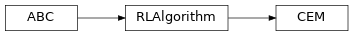# `garage.np.algos.cem`¶

Cross Entropy Method.

class CEM(env_spec, policy, sampler, n_samples, discount=0.99, init_std=1, best_frac=0.05, extra_std=1.0, extra_decay_time=100)Cross Entropy Method.

CEM works by iteratively optimizing a gaussian distribution of policy.

In each epoch, CEM does the following: 1. Sample n_samples policies from a gaussian distribution of

mean cur_mean and std cur_std.

1. Collect episodes for each policy.

2. Update cur_mean and cur_std by doing Maximum Likelihood Estimation over the n_best top policies in terms of return.

Parameters
• env_spec (EnvSpec) – Environment specification.

• policy (garage.np.policies.Policy) – Action policy.

• sampler (garage.sampler.Sampler) – Sampler.

• n_samples (int) – Number of policies sampled in one epoch.

• discount (float) – Environment reward discount.

• best_frac (float) – The best fraction.

• init_std (float) – Initial std for policy param distribution.

• extra_std (float) – Decaying std added to param distribution.

• extra_decay_time (float) – Epochs that it takes to decay extra std.

train(trainer)

Initialize variables and start training.

Parameters

trainer (Trainer) – Experiment trainer, which provides services such as snapshotting and sampler control.

Returns

The average return in last epoch cycle.

Return type

float